cancel
Showing results for
Did you mean:
See all
See all
##### Top groups
Explore all groups

## Come for the products,stay for the community

The Atlassian Community can help you and your team get more value out of Atlassian products and practices.4,365,271
Community Members

Community Events
168
Community Groups

# Calculating Value Score for Epics with Jira Align Enterprise Insights

If you have Jira Align Enterprise Insights and want to create a report that with the Value Score that is displayed on the Value tab of Epics, you may have been left scratching your head on how to do that.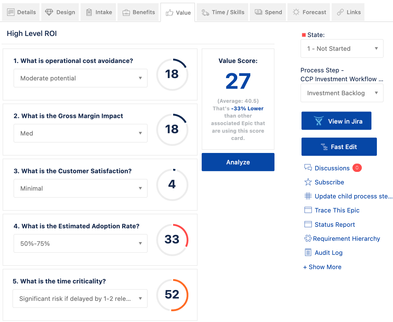The current_dw.Epic Value table within Enterprise Insights contains score value for each question answered on the Value tab for an Epic. The formula for the Value Score is relatively simple, but there is a slight catch which I will get to in a moment.

Here is the formula for Value Score:

Value Score = Average of Answer Score of all scorecard questions for an Epic

Now to the slight catch...In Jira Align, Value Score is a decimal value with one place (tenths). Here is an example of a decimal Value Score of an Epic in the UI.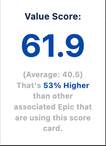The values in the Answer Score column are integer values (whole numbers).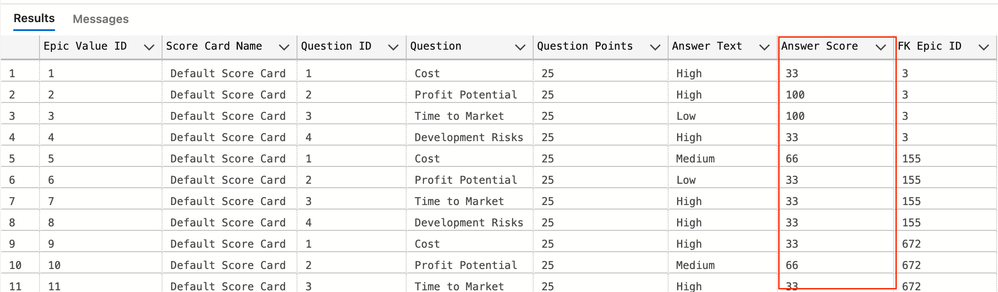If you calculate the average of an integer value, you will get an integer value in SQL and other data visualization tools. So you may need to convert the Answer Score to a decimal value first before calculating the average of the Answer Score values. Below is an example of this conversion in SQL using the CAST function. In SQL the CAST function is used twice because when the decimal Answer Score value is averaged, the resulting value is a decimal value with multiple places so it need to be converted again to a decimal value with a single place (tenths).

CAST(AVG(CAST(EV.[Answer Score] AS DECIMAL(5,1))) AS DECIMAL(5,1))

Here is an example of a SQL query that returns the value score for Epic in a portfolio.

SELECT
E.[Epic ID]
,E.[Epic Name]
,P.[Portfolio Name]
,CAST(AVG(CAST(EV.[Answer Score] AS DECIMAL(5,1))) AS DECIMAL(5,1)) AS [Value Score]
FROM current_dw.Epic AS E
INNER JOIN current_dw.[Epic Value] AS EV ON EV.[FK Epic ID] = E.[Epic ID]
INNER JOIN current_dw.Portfolio AS P ON P.[Portfolio ID] = E.[FK Portfolio ID]
WHERE
P.[Portfolio Name] = 'Domain Marketplace Pillar'
GROUP BY
E.[Epic ID]
,E.[Epic Name]
,P.[Portfolio Name]
ORDER BY
[Value Score] DESC

Below are the query results for this example.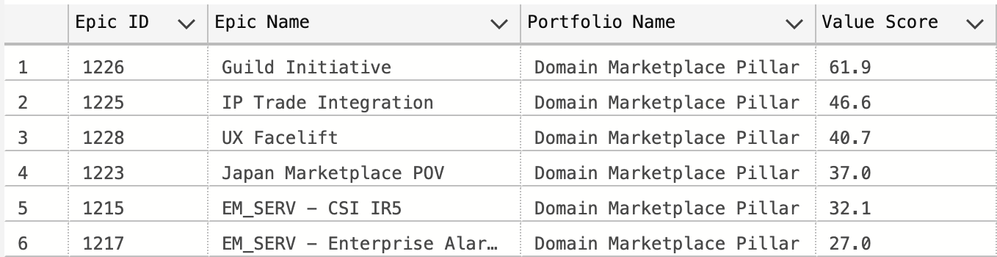Here are the value scores for the Epics in the Jira Align UI (Accessed from the Analyze button on the Value tab).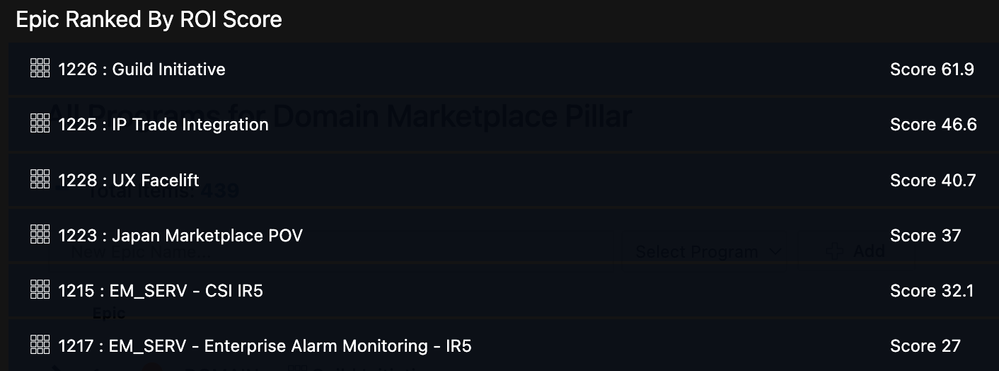As you can see, calculating the Value Score isn't a big mystery but it does requires a little transformation of the value.